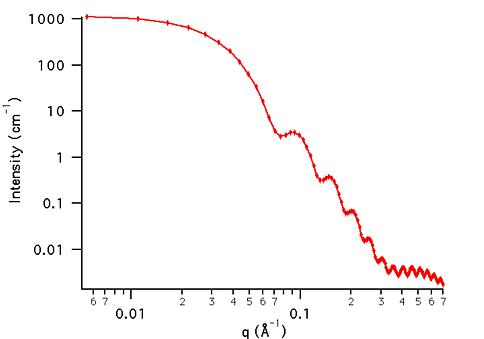MODEL

ProlateCoreShell

AUTHOR/MODIFICATION

Steve Kline 06 NOV 1998

Alan Munter 08 JULY 1999, converted to Java

APPROVED FOR DISTRIBUTION

DESCRIPTION

Calculates the form factor for a monodisperse prolate ellipsoid particle with a core/shell structure. The form factor is averaged over all possible orientations of the ellipsoid. The form factor is normalized by the total particle volume such that P(q) = scale*<f2>/Vol + bkg, where f is the single particle scattering amplitude and the < > represent the orientational average.

Resolution smeared version is also provided.

VARIABLES

Input Variables (default values):

Parameter Variable Value
0Scale1.0
5Contrast (core-shell) (Å-2)1.0e-6
6Contrast (shell-solvent) (Å-2)2.0e-6
7Incoherent Background (cm-1)0.0

USAGE NOTES

The function calculated is P(q):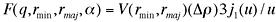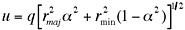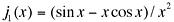The returned value is in units of [cm-1]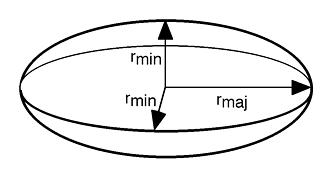Scattering contrast = SLD (core) - SLD (shell) or SLD (shell - solvent) as marked

Parameter (scale), and contrasts (Parameter and Parameter) are both multiplicative factors in the model and are perfectly correlated. No more than one of these parameters can be free during model fitting.

If the scale factor Parameter is set equal to the particle volume fraction, phi, then the returned value is the scattered intensity per unit volume, I(q) = phi*P(q). However, no interparticle interference effects are included in this calculation.

It is the users' responsibility to ensure that shell radii are larger than core radii, and that major radii are larger than minor radii.

76-point Gaussian quadrature is used to perform the orientational averaging, and may be slow on older machines.

REFERENCE

Kotlarchyk, M.; Chen, S.-H. J. Chem. Phys., 1983, 79, 2461.

Berr, S. J. Phys. Chem., 1987, 91, 4760.

TEST DATASET

This example dataset is produced by calculating the ProlateCoreShell using 128 data points, qmin = 0.001 Å-1, qmax = 0.7 Å-1 and the above default parameter values.